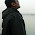### Arrange in a Sequence

Source:
Asked to me by Amol Sahasrabudhe (IITB 2004 Alumnus, Worked at Morgan Stanley Quant Division, Deutsche Bank)

Problem:
You are given 2n numbers ( 1 to n and 1 to n ). You have to arrange these numbers in a sequence such that between any two i`s , there exists exactly i-1 numbers. Is it possible for all n? If no, what are the values of n for which this is possible?

Disclaimer:
I have not been able to solve it. Sudhanshu Tungare (IITB 2008 EE Alumnus, Morgan Stanley) claims to have a solution. Cheers!

Update (November 1, 2011):
Part solution posted by Nishant Totla (CSE IITB Senior Undergraduate), Richie and Sarat in comments! Complete solution posted by Siddhant Agarwal (EE IITB Alumnus, CMI Grad student) in comments! Thanks a ton.

1.It is easy to show that such sequences cannot exist for every n. Essentially, it can be shown that a necessary condition is n ≡ 0,1 (mod 4).

Here is a proof for this:
Suppose the number i (0<i<n+1) occurs at positions xi and yi (xi<yi). Then the condition is that yi-xi = i.

From hereon, S denotes summation from i=1 to n.
So we have Syi - Sxi = Si = n(n+1)/2

But, we also have Syi + Sxi = 2n(2n+1)/2, as the yi and xi are positions in the sequence. Adding the two equations, we have 2.Syi = n(5n+3)/2.

Which gives Syi = n(5n+3)/4. Since Syi is an integer, so must be the RHS. This can happen only when n ≡ 0,1 (mod 4).

2.Building upon Nishant's work, I complete the proof by showing that there exists a sequence for number of the form n=4k,4k+1.

I will present an algorithm to construct the sequence. It will be clear to see that the sequence is properly defined and satisfies the conditions.

_ will represent unfilled elements in the sequence.
Sample case n=12,13 (for demonstration)

Case1: n=4k. (sample n=12)

Step1: Take all even numbers. Nest them together.
12,10,..,2,_,2,..,12,_,_,_,...,_

Step2: Put the biggest odd in the middle and put the other biggest odd number in the proper place on the right hand side.

12,10,..,2,11,2,..,12,_,_,..,_,11,_,..,_

Step3: We can as well now forget abt the even numbers. So we have

_,_,_,_,11,_,_,_,_,_,_

Now put the odd number which "fits" in the leftmost place such that its conjugate is just after the biggest odd number. In this case

5,_,_,_,11,5,_,_,_,_,_,_

Step4: Nest in the other odd numbers from the left

5,9,7,3,11,5,3,_,_,7,9

Step5: Put the 1's

5,9,7,3,11,5,3,1,1,7,9

DONE! (you can check that this works for all n=4k)

Case2: n=4k+1
for n=1 we have 1,1
for n=5 we have 5,1,1,3,4,5,3,2,4,2

So now assume n>=9 (Sample n=13)
This algo is almost the same as the previous algo.

Step1: Nest even numbers

12,10,..,2,_,2,..,12,_,_,_,...,_

Step2: Put biggest odd in the middle

12,10,..,2,13,2,..,12,_,_,..,_,13,_,..,_

Step3: We can as well now forget abt the even numbers. So we have

_,_,_,_,_,_,13,_,_,_,_,_,_

Now put the odd number which "fits" in the rightmost place such that its conjugate is just after the biggest odd number. In this case

_,_,_,_,_,_,13,5,_,_,_,_,5

Step4: Nest in the other odd numbers from the right

11,9,7,_,_,3,13,5,3,7,9,11,5

Step5: Put the 1's

11,9,7,1,1,3,13,5,3,7,9,11,5

DONE! (you can check that this works for all n=4k+1 with n>=9)

QED

1.Very elegant!

3.impressive sequence construction by siddhant. its elegant and also looks simple now.

4.Hi,

Another easy proof of the necessary condition.For an arrangement let f_i = no of nos between the i's , let g_i = | f_i - (i-1) |. We seek an arrangement when Sum g_i = 0; Let S = Sum g_i.

Any arrangement can be formed from 112233..nn by series of swappings. On the other hand any single swapping changes S by an even no. So an arrangement is possible only if S_0 = even. but S_0 = sigma i from 1 to n-1 = n(n-1)/2 . So this has to be even. so n =0,1 (mod 4).

5.I was reading through Richie's solution and wanted to share a solution which is a little different from his.

Let f_i be the function as defined by Richie. So sum i from 1 to n f_i=n(n-1)/2 = S(say).

When you consider any two numbers x, y, their relative arrangment in the sequence in one of the following ways:
1) ..x...y...x...y...
2) ..y...x...y...x...
3) ..y...x...x...y...
4) ..x...y...y...x...
5) ..x...x...y...y...
6) ..y...y...x...x...

Lets look at the summation S in a different way. We take a pair of numbers and see how that pair contributes to S.

lets define a function g() as the contribution to S due to the relative arrangement of the number x, y. In each of the above 6 cases the value of g will be:
1) 2
2) 2
3) 2
4) 2
5) 0
6) 0

Each of the nC2(n choose 2) pairs will contribute either 0 or 2 to S. Let 'm' be the number of pairs whose contribution is 2.
so 2m=S=n(n-1)/2
m = n(n-1)/4 which implies n is of the form 4k or 4k+1

Hope its a correct solution

6.Solution for the necessity part by Nishant Totla, Richie, Sarat are all correct. Thanks. You all basically have a combinatorial argument to count a function in two different way and since some parameters are integers, you get a constraint on n.

Solution for the construction part by Siddhant Agarwal is awesome! Thanks a lot. I was not able to reach even close.